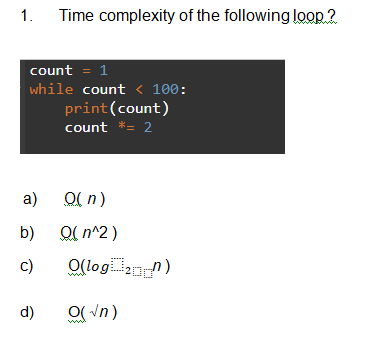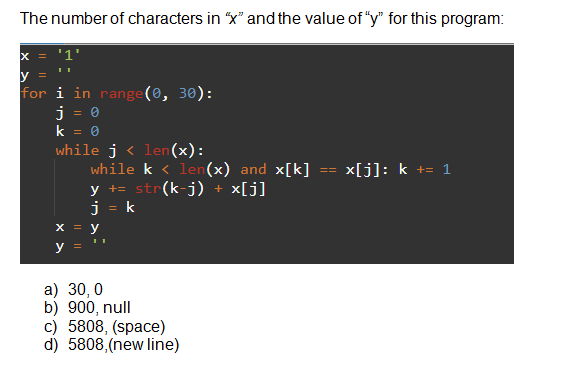# Time complexity of the following loop 21.count = 1while count < 100:print(count)count *= 2O( n)a)O( n^2)b)O(log n)c)O(Vn)d) The number of characters in “x" and the value of "y" for this program:x = '1'for i in range(0, 30):%3Dwhile j < len (x):while k < len(x) and x[k]y += str(k-j) +j = kx[j]: k += 1=D%3Dx[j]ya) 30, 0b) 900, nullc) 5808, (space)d) 5808,(new line)

Question
27 viewshelp_outlineImage TranscriptioncloseTime complexity of the following loop 2 1. count = 1 while count < 100: print(count) count *= 2 O( n) a) O( n^2) b) O(log n) c) O(Vn) d) fullscreenhelp_outlineImage TranscriptioncloseThe number of characters in “x" and the value of "y" for this program: x = '1' for i in range(0, 30): %3D while j < len (x): while k < len(x) and x[k] y += str(k-j) + j = k x[j]: k += 1 =D%3D x[j] y a) 30, 0 b) 900, null c) 5808, (space) d) 5808,(new line) fullscreen
check_circle

Step 1

For the first question, the correct option would be (C), that is, O(log­2 n).

Reason – If the variable of the loop is multiplied or divided by the constant value, then the time complexity of the lo...

### Want to see the full answer?

See Solution

#### Want to see this answer and more?

Solutions are written by subject experts who are available 24/7. Questions are typically answered within 1 hour.*

See Solution
*Response times may vary by subject and question.
Tagged in

### Coding Theory